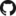# PredcompsHome

PDF Manual

Per Unit Input (original APC)

Impact (in units of output)

## Examples

Examples Overview

A fake logistic regression example predicting wine sales

A linear model with interactions

Loan defaults example

## More

As compared with Gelman & Pardoe 2007

Pairs & Weights

## Weighting Pairs of Rows

### The Original Version

In computing the APC, we assign weights to pairs of observations. Taking $$v$$ (the inputs not of interest) from the first element of the pair, $$u_1$$ from the first element of the pair, and $$u_2$$ from the second element of the pair, these samples and their weights are meant to approximate the distribution with density $$p(v)p(u_1|v)p(u_2|v)$$.

If we had many observations for each unique value of $$v$$, this would be easy: We'd just assign weight 1 to the pairs that share the same value of $$v$$, and weight 0 to the other pairs. In reality, we achieve something like this by assigning more weight to closer pairs.

The suggestion in Gelman and Pardoe 2007 is to consider all pairs of rows from the original data set and use weights based on the Mahalanobis distance $$d$$ between the corresponding $$v$$, such as $\frac{1}{1 + d}.$

### Need for Renormalizing Weights

Pairs of rows with nearby $$v$$'s have high weights, where pairs from far-away $$v$$'s have low weights. But rows with fewer $$v$$'s near them end up with less total weight than rows with more $$v$$'s near them. This isn't so good, since we meant to weight $$v$$'s according to their distribution in the original data set.

The solution is to renormalize the weights so that when grouping by the first element of each pair, the sum for each group is the same. Here's the line of code that does this in the package.

Maybe this renormalization goes without saying, but it isn't explicitly mentioned in Gelman and Pardoe 2007.

### Far Away Points Dominate

Imagine $$v$$ is a vector of $$n$$ inputs, spread uniformly throughout space. Then the expected number of points at distance $$d$$ from a given $$v$$ would be proportional to $$d^n$$. This means that using weights inversely proportional to distance will end up letting far-away points dominate, once you account for how many more far-away points there are.

### Current Implementation: Using a Fixed Number of Nearest Points

My current implementation allows you an option that addresses the above concern that far-away points dominate (in an unfortunately ad hoc manner): You can specify that for each transition start point, we keep only certain number of the nearest transition end points. This parameter is specified as onlyIncludeNearestN.

The appropriate setting for this parameter will depend on the number of points used, and maybe other properties of the data set.

### Limiting the number of points used

In the paper, Gelman and Pardoe mention that we can save on computation by, when necessary, estimating the average predictive comparison using a subset of the available data. My suggestion is that the set of points considered for transition starts need not be the same as the set considered for transition ends. For example, we choose a small subset as candidate transition starts, we may still wish to consider the full data set as potential endpoints for the transitions. This could be beneficial, since to best represent the conditional distribution $$p(u_2|v_1)$$ we're looking for $$v_2$$ close to $$v_1$$. We will find a closer $$v_2$$ by considering a larger set of potential transition endpoints than if we restricted the set of candidates for $$v_2$$ just as we restricted for $$v_1$$.

The parameters to specify are numForTransitionStart and numForTransitionEnd. They default to NULL, in which case we use the entire data set.

### Recommendations

I recommend that numForTransitionEnd should be larger than numForTransitionStart, and that onlyIncludeNearestN should be a fraction of numForTransitionEnd.

However, appropriate settings are not well understand. Please send me your feedback on what works well for you.